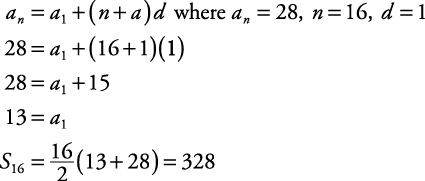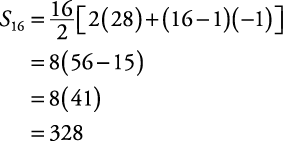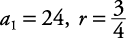## Arithmetic/Geometric Series

##### Example 1

A grocery store display of soup cans has sixteen rows with each row having one less can than the row below it. If the bottom row has twenty‐eight cans, how many cans are in the display?

This is the sum of an arithmetic sequence with sixteen terms. The sixteenth term is 28, and the common difference is 1. In order to use the formulaa 1 must be found. To do this, use the formulaThere are 328 cans in the display. Another approach could be to think of this as the sum of an arithmetic sequence with 16 terms, the first of which is a 1 = 16 and whose common difference is d = –1. Then the direct use of the equation##### Example 2

A ball is dropped from a table that is twenty‐four inches high. The ball always rebounds three fourths of the distance fallen. Approximately how far will the ball have traveled when it finally comes to rest?

Figure 1 shows this situation.

Notice that this problem actually involves two infinite geometric series. One series involves the ball falling, while the other series involves the ball rebounding.

Falling,Rebounding,Use the formula for an infinite geometric series with –1 < r < 1.The ball will travel approximately 168 inches before it finally comes to rest.

For additional review of word problems, refer to CliffsNotes Algebra I QuickReview, 2nd Edition.